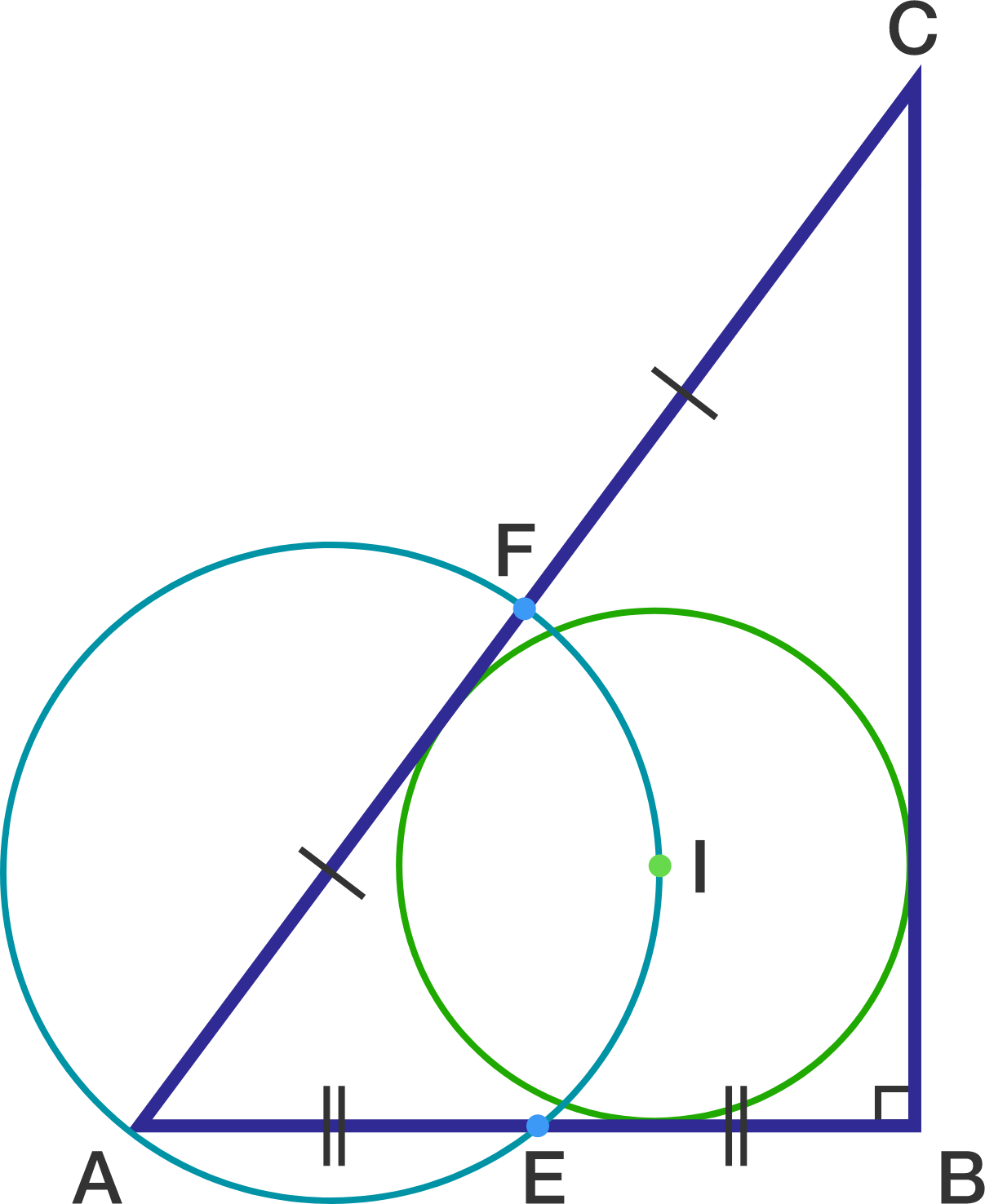# Passel Of Techniques

Geometry Level 4$ABC$ is a right triangle with $\angle B= 90^\circ$.
$E$ and $F$ are the mid-points of $AB$ and $AC$, respectively.
The incenter $I$ of triangle $ABC$ lies on the circumcircle of triangle $AEF$,

Find the ratio $\dfrac{BC}{AB}$.

If this ratio can be expressed as $\dfrac pq$, where $p$ and $q$ are coprime positive integers, submit your answer as $p+q$.

×

Problem Loading...

Note Loading...

Set Loading...